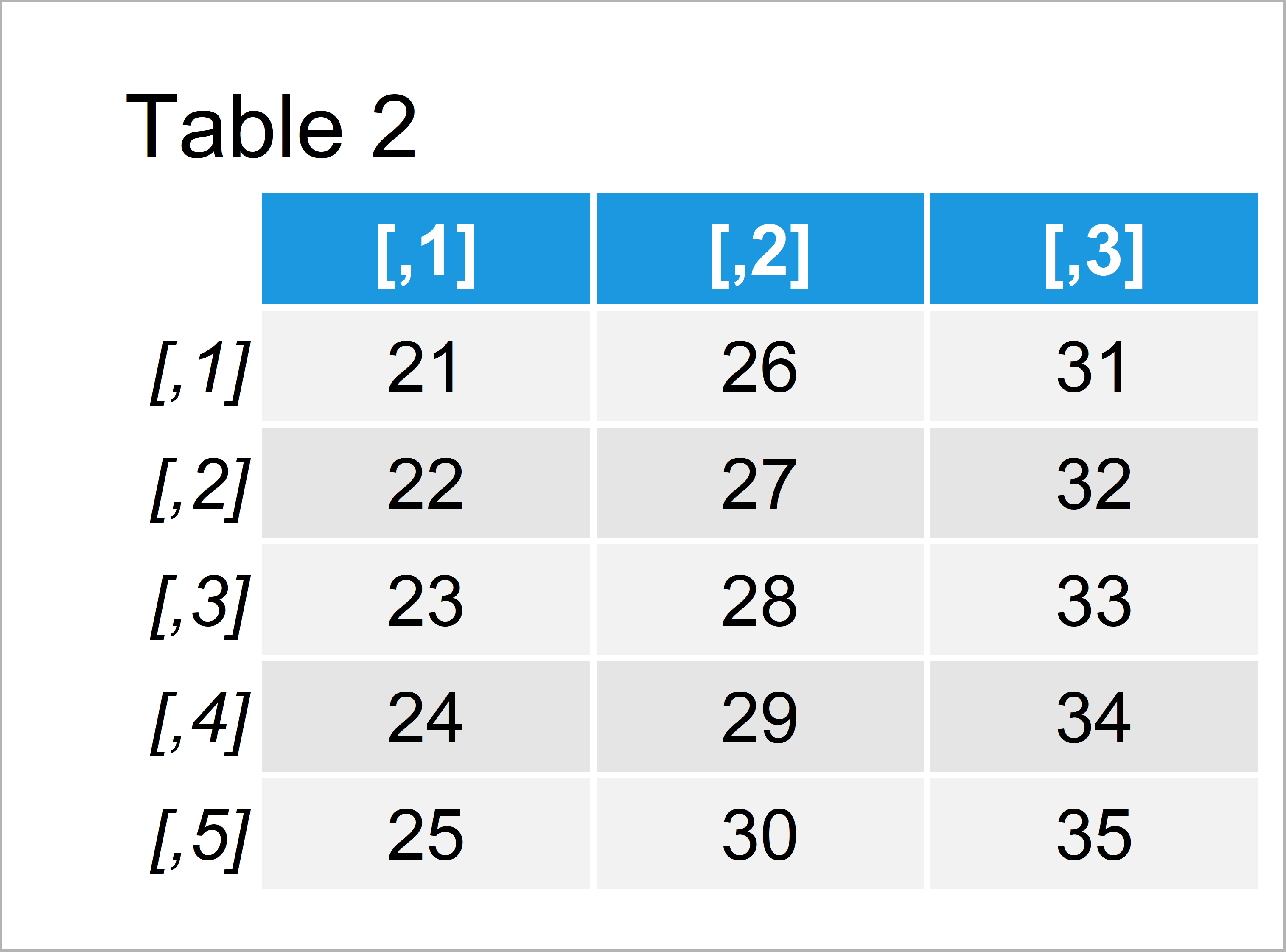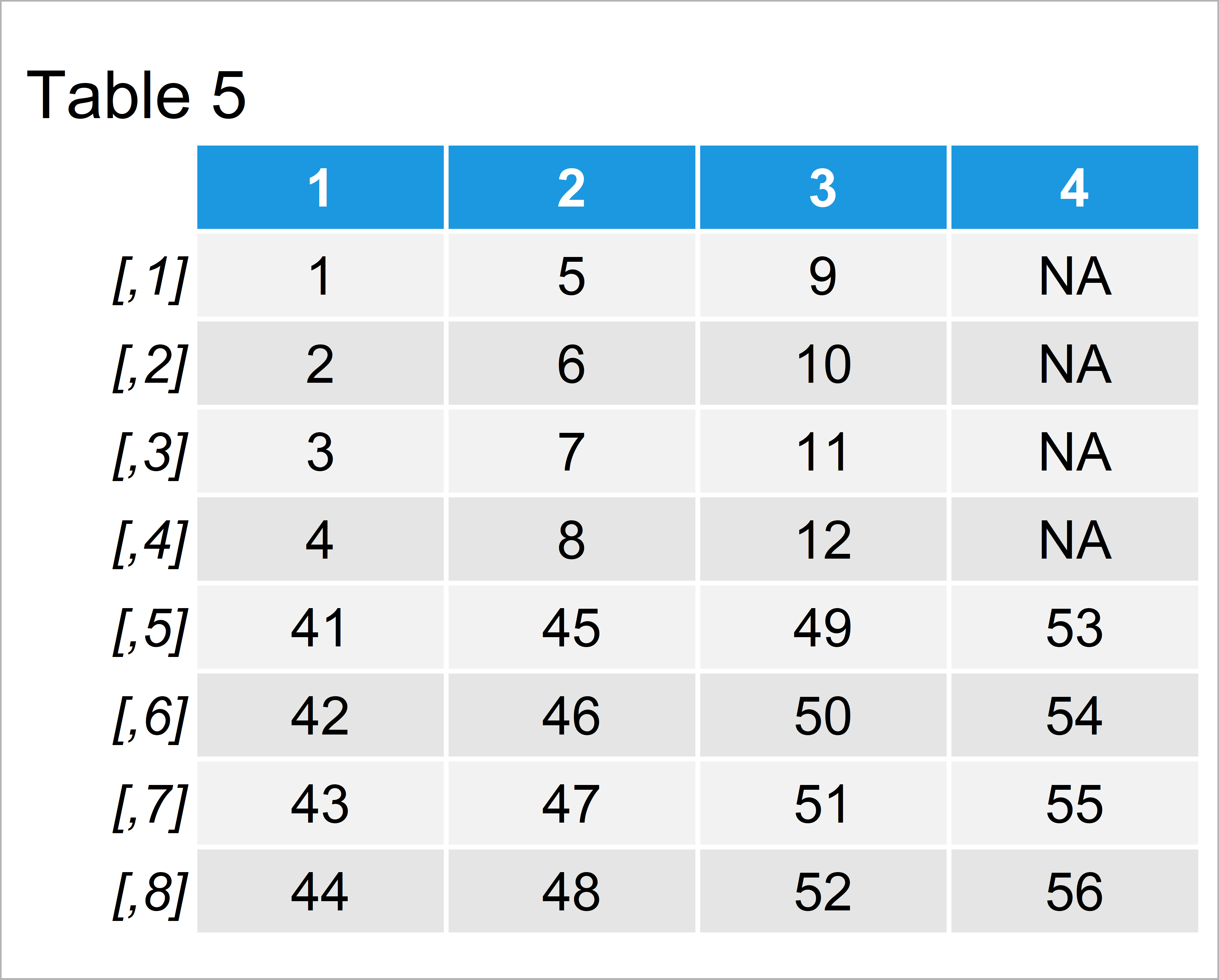# Concatenate Two Matrices in R (2 Examples)

In this tutorial, I’ll illustrate how to append two matrices in the R programming language.

The tutorial consists of these contents:

Here’s how to do it.

## Creation of Example Data

Let’s first create some example data:

```mat1 <- matrix(1:12, ncol = 3)                    # Create first example matrix
mat1                                              # Print first example matrix```As you can see based on Table 1, the first example data is a matrix and consists of four data points and three columns.

```mat2 <- matrix(21:35, ncol = 3)                   # Create second example matrix
mat2                                              # Print second example matrix```As shown in Table 2, we have created another example matrix by running the previous R syntax.

## Example 1: Append Two Matrices Using rbind() Function

In this example, I’ll explain how to concatenate the rows of the second matrix below the rows of the first matrix using the rbind function.

Consider the following R code:

```mat_combined1 <- rbind(mat1, mat2)                # Apply rbind function
mat_combined1                                     # Print concatenated matrix```In Table 3 it is shown that we have created a new matrix object containing the rows of mat1 and mat2 by running the previous code.

## Example 2: Append Two Matrices with Different Number of Columns Using rbind.fill.matrix() Function

This example explains how to bind two matrices with different columns in R.

First, we have to create another example matrix with more columns:

```mat3 <- matrix(41:56, ncol = 4)                   # Create third example matrix
mat3                                              # Print third example matrix```The output of the previous code is shown in Table 4: We have constructed a matrix with four rows and four columns. Note that our first example matrix mat1 contains only three variables.

Let’s try to join the rows of our two matrices mat1 and mat3 using the rbind function:

```rbind(mat1, mat3)                                 # rbind function leads to error
# Error in rbind(mat1, mat3) :
#   number of columns of matrices must match (see arg 2)```

As you can see, the previous R code has returned an error message to the RStudio console.

So how to fix this problem?

We first have to install and load the plyr package:

```install.packages("plyr")                          # Install plyr package

Now, we can apply the rbind.fill.matrix function of the plyr package to merge the rows of our two matrices:

```mat_combined2 <- rbind.fill.matrix(mat1, mat3)    # Apply rbind.fill.matrix function
mat_combined2                                     # Print concatenated matrix```The output of the previous code is shown in Table 5 – A new matrix consisting of the rows of mat1 and mat3.

Note that the fourth column contains NA values (i.e. missing data) in the rows that come from the input matrix mat1.

## Video & Further Resources

Do you need further explanations on the R programming codes of this article? Then you may want to have a look at the following video of my YouTube channel. In the video, I’m illustrating the R programming code of this article in a live session:

Please accept YouTube cookies to play this video. By accepting you will be accessing content from YouTube, a service provided by an external third party.If you accept this notice, your choice will be saved and the page will refresh.

Furthermore, you might want to have a look at the other tutorials on my website:

Summary: In this article, I have illustrated how to concatenate two matrix objects in the R programming language. Let me know in the comments section below, in case you have additional questions and/or comments.

Subscribe to the Statistics Globe Newsletter